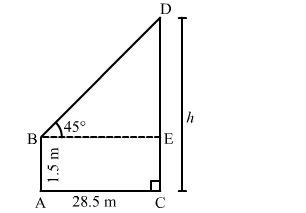# An observer 1.5 m tall 28.5 away from a tower and the angle of elevation of the top of the tower form the eye of the observer is 45°.`
Question:

An observer 1.5 m tall 28.5 away from a tower and the angle of elevation of the top of the tower form the eye of the observer is 45°. The height of the tower is

(a) 27 m
(b) 30 m
(c) 28.5 m
(d) none of these

Solution:

(b) 30 m

Let $A B$ be the observer and $C D$ be the tower.Draw $B E \perp C D$, Let $C D=h$ metres. Then,

$A B=1.5 \mathrm{~m}, B E=A C=28.5 \mathrm{~m}$ and $\angle E B D=45^{\circ}$.

$D E=(C D-E C)=(C D-A B)=(h-1.5) \mathrm{m} .$

In right $\Delta B E D$, we have:

$\frac{D E}{B E}=\tan 45^{\circ}=1$

$\Rightarrow \frac{(h-1.5)}{28.5}=1$

$\Rightarrow h-1.5=28.5$

$\Rightarrow h=28.5+1.5=30 \mathrm{~m}$

Hence the height of the tower is $30 \mathrm{~m}$.